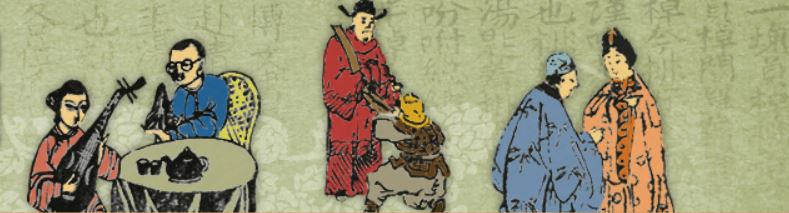#•

## 最新懺悔的歌隨堂測驗• （a） 著名作品如〈最後一夜〉、〈意難忘〉。
• （b） 〈最新懺悔的歌〉以「五言律詩」的形體撰寫。
• （c） 為日治時期臺語流行樂界重要人物之一。

• （a） 譜有〈月夜愁〉一曲。
• （b） 著名作品如〈雨夜花〉、〈望春風〉。
• （c） 善於彈奏洋琴。

• （a） 《戒嫖新歌三婿祝壽最新倡門賢母最新懺悔的合歌》
• （b） 《最新解勸盤賭歌》
• （c） 《雪梅思君歌》

• （a） 陳美珠。
• （b） 陳寶貴。
• （c） 楊麗花。

• （a） 為日治時期新曲。
• （b） 為傳統唸唱使用歌調。
• （c） 蘇桐作曲，井田一郎編曲。

• （a） 為電影《懺悔》於臺灣上映時的宣傳曲。
• （b） 未承襲傳統七字仔形式，不強調歌詞排比和工整。
• （c） 李臨秋作詞。

• （a） 敘述男子因留連花街柳巷，敗光家產而後悔莫及。
• （b） 敘述李妙英為撫養孩子落入風塵。
• （c） 敘述梅氏一馬兩鞍，用情不專，最後懺悔遁入空門。

• （a） 喻一女嫁二夫。
• （b） 意同「從一而終」
• （c） 意同「一馬難將兩鞍鞴」。

• （a） 屎緊掘屎礐。
• （b） 要嫁才要縛腳。
• （c） 好天也著積雨來糧。

• （a） 「花再開」形容梅氏入佛門，求得心情平靜。
• （b） 「牡丹當開糖蜜甜」形容女人青春年華，如牡丹盛開。
• （c） 「花落無人相看見」形容青春不再，不再受關注。